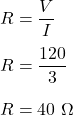## 3. A PS6 plugged into a 120 V outlet uses 3 Amps. What is the Resistance rating of the power cord? voltage: V= current: 1

Question

3. A PS6 plugged into a 120 V outlet uses 3 Amps. What is the Resistance rating of
the power cord?
voltage: V=
current: 1 =
resistance: R=
V=IxR
1=VR R
Underline/Bold Which Equation we are going to use:
= V
Plug in the numbers from above and solve for our answer:

in progress 0
7 months 2021-07-30T04:29:35+00:00 1 Answers 2 views 0

R = 40 ohms

Explanation:

Given that,

The voltage of outlet, V = 120 V

Current flowing through the device, I = 3 A

We need to find the Resistance rating of  the power cord. Let the resistance is R. We know that,

Ohm’s law, V = IR

Put all the values,So, the resistance rating of the power cord is equal to 40 ohms.